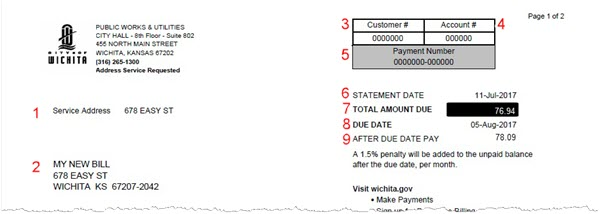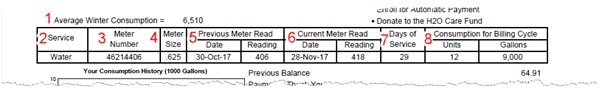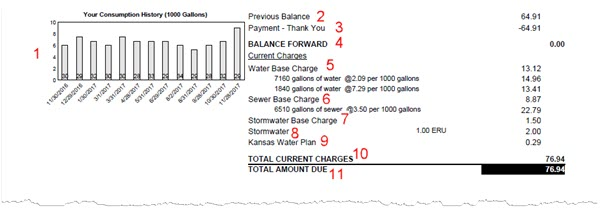# Residential Water Bill: How to Read - Example and Explanations

To help understand a residential water bill, an example bill has been divided into three sections below, with each section showing a portion of the bill followed by details about each item in that section.

Section 1:2. [Addressed to] the primary account holder and water bill mailing address.
3. Customer # is the record number of the primary account holder.
4. Account # is the number of the service address.
5. Payment # is the complete account number used to record payments accurately.
6. STATEMENT DATE is the date that the current bill was created.
7. TOTAL AMOUNT DUE is the total amount currently due on the account.
8. DUE DATE is the date by which the total amount should be paid to avoid late penalties.
9. AFTER DUE DATE PAY: the total amount to pay if payment is made after the due date. The amount shown is valid during the first month after the due date. The balance due increases by 1.5% per month if unpaid.

Section 2:1. Average Winter Consumption (AWC) is the average gallons of water used at the property from December through March, with a minimum of 6000 gallons for the average residential property.
2. Service is the utility being provided (Water).
3. Meter Number is the number of the meter that measured the water usage.
4. Meter Size is the meter water pipe size. The meter size is used as part of the rate calculation. The average residential meter size is .625 inches.
5. Previous Meter Read includes the date and total units of water usage from the previous bill’s meter reading. One unit equals 750 gallons of water.
6. Current Meter Read includes the date and total units of water usage from the current bill’s meter reading.
7. Days of Service is the number of days in between the previous and current bill’s meter reading.
8. Consumption for Billing Cycle is the total amount of water used during the current billing cycle. Units is the number of units of water used between the current and previous meter reading. Gallons is the total number of gallons of water used for the current billing cycle.

Section 3:1. Your Consumption History includes a chart showing water usage totals for the past year. The dates listed along the bottom of the chart are the billing dates and the numbers along the left side of the chart represents thousands of gallons. Each shaded column represents the gallons of water used during each billing cycle. The number at the base of each column is the “Days of Service” for each billing date (higher usage for a billing cycle may be due to a higher number of days of service in that billing cycle).
2. Previous Balance is the total amount due on the previous month’s bill.
3. Payment is the total amount paid since the previous month’s bill.
4. Balance Forward is the difference between the previous balance and payment totals.
5. Water Base Charge is the monthly amount charged for water service based on the meter size. The base charge is applied whenever water service is available during the billing cycle, regardless of whether any water is used.

Below the water base charge are up to three lines showing consumption (usage) charges. Each line represents a "tier" or "block" of usage.

• The first line, tier/block 1, shows the total volume of water used up to 110% of the Average Winter Consumption (see explanation of Average Winter Consumption above) and the charge for that total.
• The second line, tier/block 2, shows the total volume of water used between 111% and 310% of the Average Winter Consumption and the charge for that total.
• The third line, tier/block 3, shows the total volume of water used above 311% of the Average Winter Consumption and the charge for that total.
• As an example: if the Average Winter Consumption is 6,000 gallons, all usage up to 110% (6,600 gallons) will be charged at the first tier/block rate. The next 200% (12,000 gallons) will be charged at the second tier/block rate. All usage above 18,601 gallons will be charged at the third tier/block rate.
• The current tier/block rates can be found here (select the "Rate Ordinance - Water" link for the current year): Water Rates
1. Sewer Base Charge is the monthly amount charged for sewer service and is based on the water meter size. Below the sewer base charge is a line showing the sewer usage charge. The usage charge is based on the total amount of water used at the property during the billing cycle.
2. Stormwater Base Charge is the monthly amount charged for stormwater runoff maintenance.
3. Stormwater is the charge based on the property’s number of ERUs (Equivalent Residential Units). One ERU equals 2,139 square feet of impervious surface.
4. Kansas Water Plan is the fee charged by the state for the Kansas Water Plan.
5. Total Current Charges is the total amount for the current billing cycle.
6. Total Amount Due is the total amount currently due on the account.

For additional billing information or assistance, please email watercustomerservice@wichita.gov or call 316-265-1300.